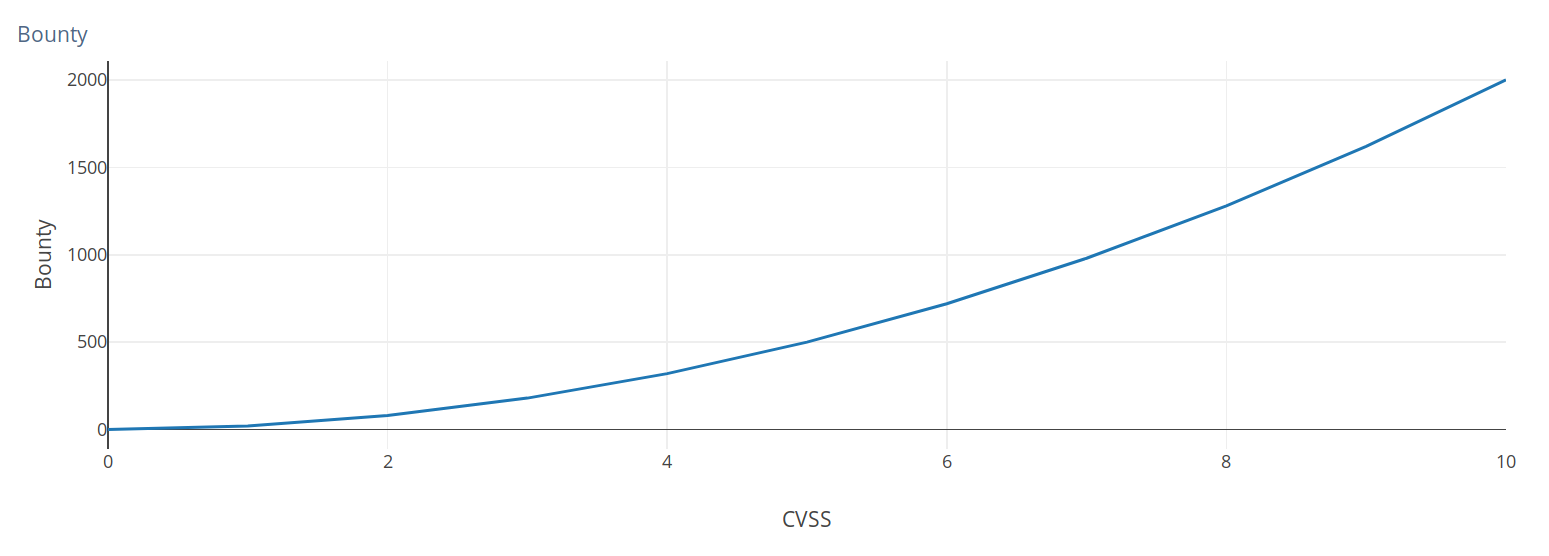# The math behind bug bounties — A formula to calculate bounty amounts.

Nov 29, 2017

Inspired by Daniel Adams’ work, Tom Hudson and I have assembled a formula to calculate the final bounty amount based on the impact of the reported security vulnerability. The formula can be expressed as follows where $b$ is the bounty amount, $C$ is the CVSS score, and $N$ is a constant that creates a direct correlation between $b_{max}$ and $C_{max}$:

In pseudo-code this could be expressed as follows:

// Input: b_max, C, and n
// Output: b

function formula(b_max, C, n)
if C > 10 or C < 0 then
return error
else if n < 1 or n > 3 then
return error
else
C_max := 10
N := b_max / C_max^n
b := N * C^n
return b
end if
end function


$C_{max}$ is always $10$ and $b_{max}$ is the maximum bounty amount. $b_{max}$ and $n$ are the only variables which the bug bounty program needs to define for general purposes. $n$ is in the set of the positive real numbers ($\Bbb{R}^+$). We recommend setting a lower value for $n$ as you increase $b_{max}$. This should ensure that the gap between values in the 7.0 to 10.0 CVSS score range is not too big.

An exponent $n$ greater than $1$ creates an exponential curve as seen in the graph below:Figure 1: Plot graph for $b_{max} = 2000$ and $n = 2$.

Some programs reward based on the Qualitative Severity Rating  and not the exact CVSS score. This can easily be achieved by always rounding the value up to the maximum score of the corresponding CVSS rating.

It is important to note that we are using CVSS as an example system and that this formula could be adapted to use any vulnerability scoring system. That said, we are assuming that the reader is using all three metric groups of the CVSS system when evaluating the total severity score.  Platforms such as HackerOne do not use all three groups when evaluating the final score.

This is a little calculator based on our formula. You can supply it with different values and inspect the results below.

After spending some time playing around with this formula, we became aware of how it could be used in various areas of the bug bounty industry. This write-up highlights the potential benefits of using our proposed mechanism.

## Transparency

Bug bounty programs can now place this formula in their security policy and make it clear how they came to the various bounty amounts. We will describe further on how platforms could aid with this process.

## Ease of use

Our proposed formula requires very few steps to set up. Bug bounty programs are only required to define two variables, $b_{max}$ and the exponent $n$.

## Capping

This approach also enables programs to cap the curve at any desired point. HackerOne, for instance, requires a \$50 minimum bounty. So if the rate starts to drop below that, one can simply remove everything below \$50.

## How this could be implemented by bug bounty platforms

HackerOne currently suggest bounty amounts when a program wants to set a reward for the researcher after triaging a report. Their current approach is statistical and focuses on competitive bounty amounts. The numbers are generated based on the average bug bounty payouts for the corresponding CVSS score on HackerOne’s platform.

The bounty amount is currently broken down into three categories median, competitive, and top. We believe it is possible to replace these values with the exponent $n$. In other words, the median category could mean a value of $3$ for $n$, and top could be $n = 1$. The exponent allows the program to evaluate a bounty amount based on the relative impact of the various CVSS scores. A more mature program that wants to offer competitive bounty amounts can set a lower value for $n$, which in turn means the gap between the individual bounty amounts would be smaller.

On top of having bounty amounts suggested in the payment process, we encourage platforms to have an inbuilt calculator that automatically inserts all values for the different CVSS scores in a table. This dynamic and efficient approach makes it easier for programs to quickly set up a bounty table in their policy and update it in the future.

## Conclusion

In the hopes of seeing more programs and platforms adopt this formula, we are open sourcing everything including the source code for the calculator on GitHub. The repository is located here. We welcome any contributions and feedback from the public.

Update (Friday, 01 December 2017): @richinseattle noticed that values greater than $2$ for $n$ create very significant gaps between CVSS scores in the high and critical range. There is a ticket to keep track of this issue on GitHub and we hope to figure out a solution very soon: https://github.com/EdOverflow/bounty-formula/issues/3.# Support my work

If you enjoy reading my write-ups and would like to support my work,